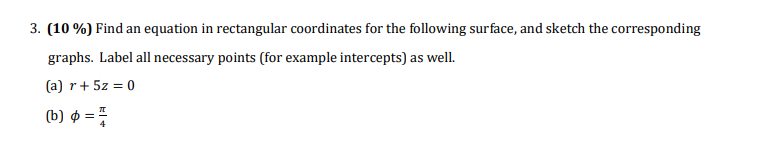Home / Answered Questions / Other / 3-10-find-an-equation-in-rectangular-coordinates-for-the-following-surface-and-sketch-the-correspond-aw198

# (Solved): 3. (10%) Find An Equation In Rectangular Coordinates For The Following Surface, And Sketch The Corre...3. (10%) Find an equation in rectangular coordinates for the following surface, and sketch the corresponding graphs. Label all necessary points (for example intercepts) as well. (a) r+ 5z = 0 b) 0 = 1

We have an Answer from Expert